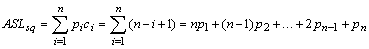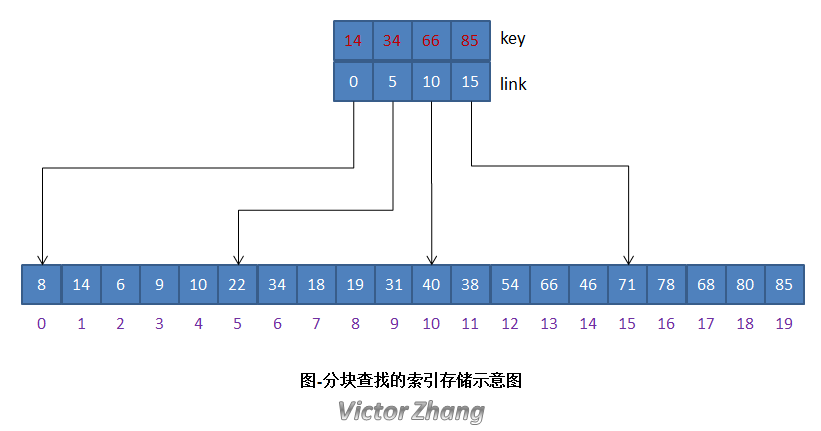# 查找的基本概念

——平均查找长度ASL（Average Search Length）(1) 使用什么数据存储结构（如线性表、树形表等）。

(2) 表中的次序，即对无序表还是有序表进行查找。

# 顺序查找

public int orderSearch(int[] list, int length, int key) {
// 从前往后扫描list数组，如果有元素的值与key相等，直接返回其位置
for (int i = 0; i < length; i++) {
if (key == list[i]) {
return i;
}
}

// 如果扫描完，说明没有元素的值匹配key，返回-1，表示查找失败
return -1;
}

ASL = (N + N-1 + ... + 2 + 1) / N = (N+1) / 2

# 二分查找

(1) 必须是顺序存储结构。

(2) 必须是有序的表。

public int binarySearch(int[] list, int length, int key) {
int low = 0, mid = 0, high = length - 1;
while (low <= high) {
mid = (low + high) / 2;
if (list[mid] == key) {
return mid; // 查找成功，直接返回位置
} else if (list[mid] < key) {
low = mid + 1; // 关键字大于中间位置的值，则在大值区间[mid+1, high]继续查找
} else {
high = mid - 1; // 关键字小于中间位置的值，则在小值区间[low, mid-1]继续查找
}
}
return -1;
}

# 分块查找(1) 首先查找索引表

(2) 然后在已确定的块中进行顺序查找1 public class BlockSearch {
2
3     class IndexType {
4         public int key; // 分块中的最大值
5         public int link; // 分块的起始位置
6     }
7
8     // 建立索引方法，n 是线性表最大长度，gap是分块的最大长度
9     public IndexType[] createIndex(int[] list, int n, int gap) {
10         int i = 0, j = 0, max = 0;
11         int num = n / gap;
12         IndexType[] idxGroup = new IndexType[num]; // 根据步长数分配索引数组大小
13
14         while (i < num) {
15             j = 0;
16             idxGroup[i] = new IndexType();
17             idxGroup[i].link = gap * i; // 确定当前索引组的第一个元素位置
18             max = list[gap * i]; // 每次假设当前组的第一个数为最大值
19             // 遍历这个分块，找到最大值
20             while (j < gap) {
21                 if (max < list[gap * i + j]) {
22                     max = list[gap * i + j];
23                 }
24                 j++;
25             }
26             idxGroup[i].key = max;
27             i++;
28         }
29
30         return idxGroup;
31     }
32
33     // 分块查找算法
34     public int blockSearch(IndexType[] idxGroup, int m, int[] list, int n, int key) {
35         int mid = 0;
36         int low = 0;
37         int high = m -1;
38         int gap = n / m; // 分块大小等于线性表长度除以组数
39
40         // 先在索引表中进行二分查找，找到的位置存放在 low 中
41         while (low <= high) {
42             mid = (low + high) / 2;
43             if (idxGroup[mid].key >= key) {
44                 high = mid - 1;
45             } else {
46                 low = mid + 1;
47             }
48         }
49
50         // 在索引表中查找成功后，再在线性表的指定块中进行顺序查找
51         if (low < m) {
52             for (int i = idxGroup[low].link; i < idxGroup[low].link + gap; i++) {
53                 if (list[i] == key)
54                     return i;
55             }
56         }
57
58         return -1;
59     }
60
61     // 打印完整序列
62     public void printAll(int[] list) {
63         for (int value : list) {
64             System.out.print(value + " ");
65         }
66         System.out.println();
67     }
68
69     // 打印索引表
70     public void printIDX(IndexType[] list) {
71         System.out.println("构造索引表如下：");
72         for (IndexType elem : list) {
74         }
75         System.out.println();
76     }
77
78     public static void main(String[] args) {
79         int key = 85;
80         int array[] = { 8, 14, 6, 9, 10, 22, 34, 18, 19, 31, 40, 38, 54, 66, 46, 71, 78, 68, 80, 85 };
81         BlockSearch search = new BlockSearch();
82
83         System.out.print("线性表: ");
84         search.printAll(array);
85
86         IndexType[] idxGroup = search.createIndex(array, array.length, 5);
87         search.printIDX(idxGroup);
88         int pos = search.blockSearch(idxGroup, idxGroup.length, array,
89                 array.length, key);
90         if (-1 == pos) {
91             System.out.format("查找key = %d失败", key);
92         } else {
93             System.out.format("查找key = %d成功，位置为%d", key, pos);
94         }
95     }
96
97 }

key = 14, link = 0
key = 34, link = 5
key = 66, link = 10
key = 85, link = 15

# 三种线性查找的PK

(1) 以平均查找长度而言，二分查找 > 分块查找 > 顺序查找。

(2) 从适用性而言，顺序查找无限制条件，二分查找仅适用于有序表，分块查找要求“分块有序”。

(3) 从存储结构而言，顺序查找和分块查找既可用于顺序表也可用于链表；而二分查找只适用于顺序表。

(4) 分块查找综合了顺序查找和二分查找的优点，既可以较为快速，也能使用动态变化的要求。

# 参考资料

《数据结构习题与解析》（B级第3版）

# 相关阅读

posted @ 2015-03-10 18:29  静默虚空  阅读(9307)  评论(1编辑  收藏  举报